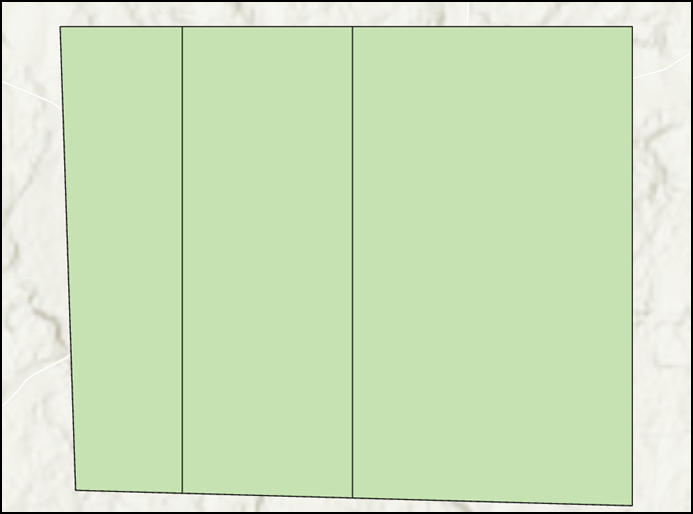English
Continue in the app
Be The First To Get Support Updates
Want to know about the latest technical content and software updates?

# How To: Split a polygon by percentage via ArcPy in ArcGIS Pro

## Summary

ArcGIS Pro allows splitting a polygon feature into several segments by using tools such as the Split (Analysis) tool and the Split tool from the Modify Features pane. However, the tools provided are unable to split the polygon by percentage. Follow the steps provided in this article to split a polygon by percentage in the Python window.

## Procedure

1. Import the ArcPy module and configure the environment of the script.
```Import arcpy
p = arcpy.mp.ArcGISProject("CURRENT")
m = p.listMaps("<Map_Name>")
lyr = m.listLayers(0)
```
1. Specify the polygon split percentage using an array parameter. In this example, the polygon is split by 20%, 30%, and 50%.
```splits = [20, 30, 50]
```
1. Retrieve the polygon and the polygon extent properties using the SearchCursor function.
```with arcpy.da.SearchCursor("<Layer_Name>", ["SHAPE@"]) as pcursor:
for prow in pcursor:
polygon = prow    # polygon to cut
e = polygon.extent   # bounding extent of polygon
print(e.XMin,e.YMin,e.XMax,e.YMax)
del pcursor
```
1. Create a variable to store the geometric iteration accuracy value.
```stepsize = 0.001
```
1. Specify the parameters to set the polygon split direction.
```leftXstart = e.XMin
leftX = e.XMin + stepsize
ymax = e.YMax
ymin = e.YMin
```
1. Iterate through the polygon cut process using the cut() function according to the split percentages set in Step 2.
```cutpoly = polygon
icursor = arcpy.da.InsertCursor("<Layer_Name>", ["SHAPE@"])
for i in splits[:2]:
print(i)
tol = 0
while tol < i:
# construct NS line
pntarray = arcpy.Array()
pline = arcpy.Polyline(pntarray,arcpy.SpatialReference(<WKID>))
# cut polygon and get split-parts
cutlist = cutpoly.cut(pline)
tol = 100 * cutlist.area / polygon.area
leftX += stepsize
#print str(leftX) + ":" + str(tol)
cutpoly = cutlist
icursor.insertRow([cutlist])
# part 0 is on the right and part 1 is on the left of the split
```
1. Insert the last split remainder.
```icursor.insertRow([cutlist])
del icursor
```

The code below shows the full working script.

```Import arcpy
p = arcpy.mp.ArcGISProject("CURRENT")
m = p.listMaps("Map")
lyr = m.listLayers(0)
splits = [20, 30, 50]
with arcpy.da.SearchCursor("testpolygon", ["SHAPE@"]) as pcursor:
for prow in pcursor:
polygon = prow
e = polygon.extent
print(e.XMin,e.YMin,e.XMax,e.YMax)
del pcursor
stepsize = 0.001
leftXstart = e.XMin
leftX = e.XMin + stepsize
ymax = e.YMax
ymin = e.YMin
cutpoly = polygon
icursor = arcpy.da.InsertCursor("testpolygon", ["SHAPE@"])
for i in splits[:2]:
print(i)
tol = 0
while tol < i:
pntarray = arcpy.Array()
pline = arcpy.Polyline(pntarray,arcpy.SpatialReference(3857))
cutlist = cutpoly.cut(pline)
tol = 100 * cutlist.area / polygon.area
leftX += stepsize
cutpoly = cutlist
icursor.insertRow([cutlist])
icursor.insertRow([cutlist])
del icursor
```

The image below demonstrates the polygon split by 20%, 30%, and 50% after running the Python script in ArcGIS Pro.## Related Information

Last Published: 8/15/2022

Article ID: 000027999

Software: ArcGIS Pro 3.0.1, 3.0, 2.9.4, 2.9.3, 2.9.2, 2.9.1, 2.9, 2.8.7, 2.8.6, 2.8.5, 2.8.4, 2.8.3, 2.8.2, 2.8.1, 2.8